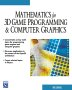# Maths - Matrix Factoring

It is very useful to be able divide up a single matrix into several matrices multiplied together. For instance if a matrix represents a transform it is often useful to replace it with a sequence of simpler transforms. This may not seem like the most exciting subject but since we are interested in transforms there are some useful applications.

## Applications

Applications include:

### Matrix to Euler

If we have a rotation matrix we can factor this into 3 separate rotations around the x,y and z axis (or heading, attitude and bank). This is discussed on this page.

### Decompose into Rotate, Scale, Shear, Reflect, etc.

I don't know if this is possible, but it would be very useful if we could take any arbitrary matrix representing a transform and factor it into rotate, scale, shear, reflect, etc. components. An example of this sort of thing is shown on this page.

### Square Root

If we can divide a matrix [m1] into two equal factors, this gives the square root.

[m1] = [m2][m2]

[m1] = [m2]²

√[m1] = [m2]

### Reothorthgonalising a Matrix

When we are working with matrices representing rotations with an orthogonal basis then, after some operations, the matrices can become slightly de-orthgonalised due to small rounding errors. We need an algorithm to correct this and reothorthgonalise the matrix. There are various possible methods of doing this, discussed on this page, This methods can involve factoring the matrix into orthogonal and non-orthogonal components similar to the SVD method below.

### Simplifying an Inertia Tensor

The Inertia Tensor in 3D is a matrix, by choosing suitable local coordinates for the solid body we can make the non-diagonal elements of the matrix zero, in other words, a matrix which only multiplies in the x, y and z directions. For more general local coordinates the inertia tensor is equivalent to the following factorisation:

• A rotation matrix which aligns the eigenvectors with the x, y and z coordinates
• A diagonal matrix which only multiplies in the x, y and z directions
• A rotation matrix which is the inverse of the first and restores the coordinates.

## General Methods

Some of the general methods for factoring a matrix are:

### Spectrial or Eigen Decomposition

This factors a matrix into rotation and diagonal matrices but it only works for certain types of matrix, specifically 'normal matricies'.

[M] = [U][D][U]t

where:

• [M] = a 'normal matrix' to be factored
• [U] = a rotation matrix
• [D] = a diagonal matrix (non-diagonal terms are zero)

A 'normal matrix' is a normal operator in Hilbert space. A normal matrix is defined by:

[M][M]* = [M]*[M]

where:

Calculating the factors:

[U] = To calculate the rotate matrix it is made up from the eigenvectors of the matrix.

[D] = To calculate the diagonal terms, they are the eigenvalues in the same order as the corresponding eigenvectors in [U].

### SVD (Singular Value Decomposition)

This is a more general version of the eigen decomposition above. This works on any matrix including non-square matrices.

[M] = [U][D][V]t

where:

• [M] = a matrix to be factored
• [U] = a matrix made up of a set of orthogonal 'output' vectors.
• [V] = a matrix made up of a set of orthogonal 'input' vectors.
• [D] = a diagonal matrix (non-diagonal terms are zero)

The calculation of [U],[D] and [V] involves a complex algorithm, code to implement it can be found in this project Open Computer Vision Library.

 metadata block see also: Correspondence about this page david Book Shop - Further reading. Where I can, I have put links to Amazon for books that are relevant to the subject, click on the appropriate country flag to get more details of the book or to buy it from them.Mathematics for 3D game Programming - Includes introduction to Vectors, Matrices, Transforms and Trigonometry. (But no euler angles or quaternions). Also includes ray tracing and some linear & rotational physics also collision detection (but not collision response). Specific to this page here:

This site may have errors. Don't use for critical systems.